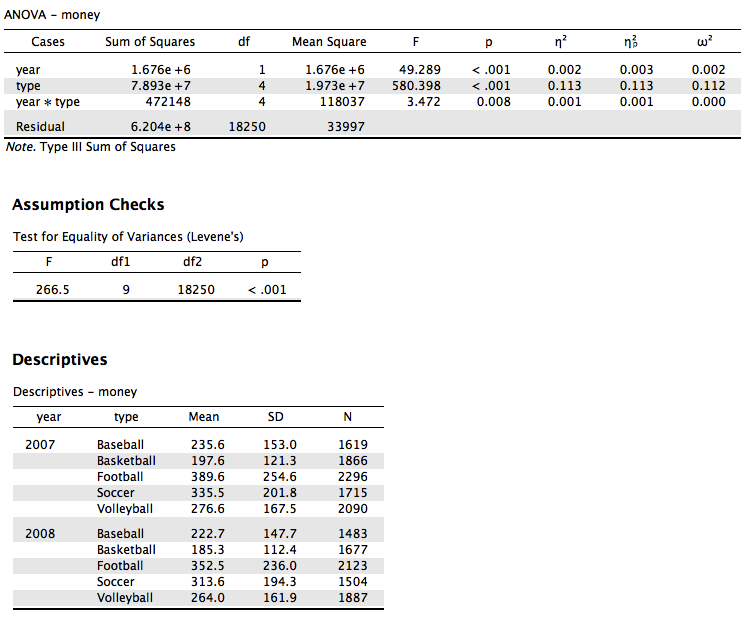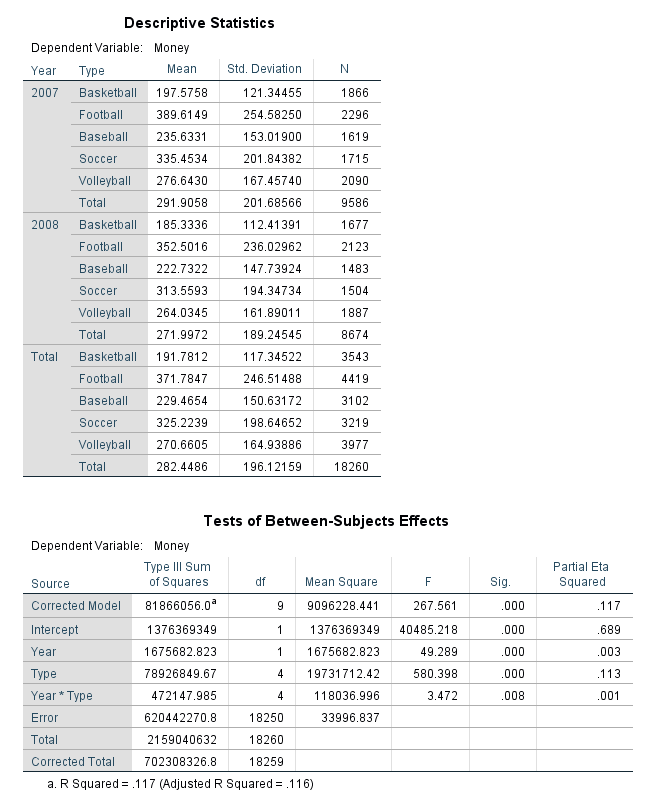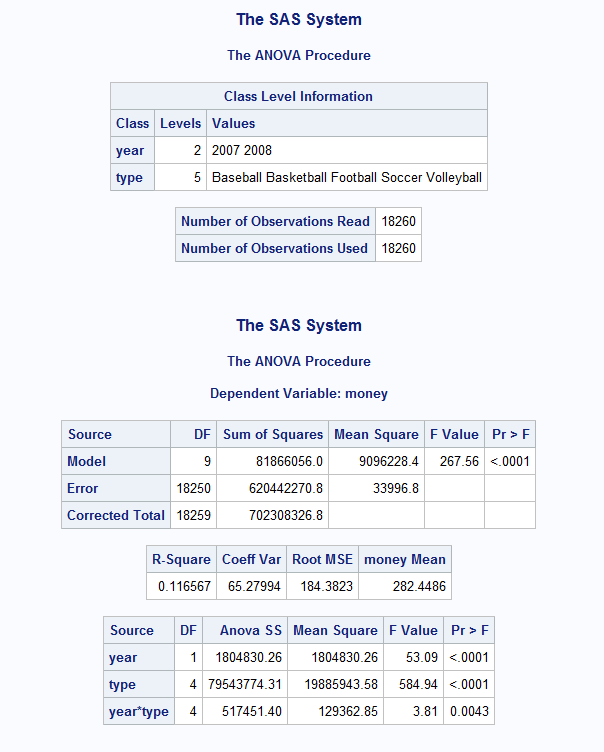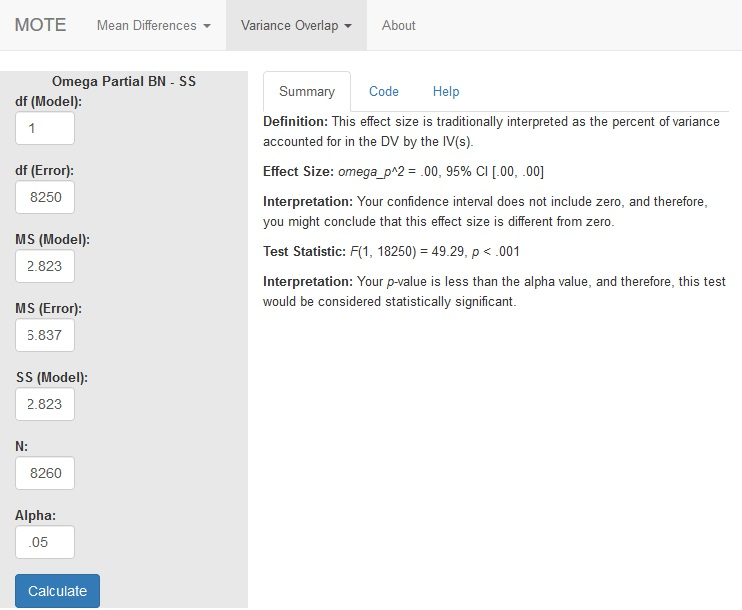# Description

This function displays omega squared from ANOVA analyses and its non-central confidence interval based on the F distribution. This formula is appropriate for multi-way between subjects designs.

The formula for $\omega_p^2$ is: $$\frac{df_{model} \times (MS_{model} - MS_{error})}{SS_{model} + (N - df_{model}) \times MS_{error}}$$

The formula for F is: $$\frac{MS_{model}}{MS_{error}}$$

# R Function

omega.partial.SS.bn(dfm, dfe, msm, mse, ssm, n, a)

# Arguments

• dfm = degrees of freedom for the model/IV/between
• dfe = degrees of freedom for the error/residual/within
• msm = mean square for the model/IV/between
• mse = mean square for the error/residual/within
• ssm = sum of squares for the model/IV/between
• n = total sample size
• a = significance level

# Example

We looked at two year’s worth of athletic spending data (treating each receipt and years as separate between subjects’ events) for four different sports. Are there differences across sports and years in spending?

JASPSPSSSAS# Function in R:

omega.partial.SS.bn(dfm = 1, dfe = 18250, msm = 1675682.823, mse = 33996.837, ssm = 1675682.823, n = 18260, a = 0.05)

# MOTE

## Screenshot## Effect Size:

$\omega_p^2$ = .00, 95% CI [.00, .00]

## Interpretation:

Your confidence interval does not include zero, and therefore, you might conclude that this effect size is different from zero.

Not applicable.

## Test Statistic:

F(1, 18250) = 49.29, p < .001

## Interpretation:

Your p-value is less than the alpha value, and therefore, this test would be considered statistically significant.